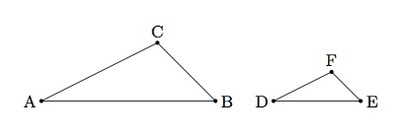Defining Trigonometric Ratios

Alignments to Content Standards: G-SRT.C.6

Below is a picture of $\triangle ABC$:1. Draw a triangle $DEF$ that is similar (but not congruent) to $\triangle ABC$.

2. How do $\frac{|DE|}{|DF|}$ and $\frac{|AB|}{|AC|}$ compare? Explain.

3. When $\angle B$ is a right angle, the ratio $|AB|:|AC|$ is called the cosine of $\angle A$ while the ratio $|BC|:|AC|$ is called the sine of $\angle A$. Why do these ratios depend only on $\angle A$?

4. The ratios in part (c) make sense whether or not $\angle B$ is a right angle but they are only given names (sine and cosine) in this special case. What is special about the case where $B$ is a right angle?

IM Commentary

The purpose of this task is to use the notion of similarity to define the sine and cosine of an acute angle. When two triangles are similar triangles ratios of their corresponding side lengths are equivalent. In the special case when the triangles in question are right triangles and the ratio is that of a leg to the hypotenuse, these ratios depend, up to equivalence, only on the (acute) angle measures of the triangle. These equivalent ratios provide the definition of the sine and cosine of any acute angle.

The task first investigates ratios of side lengths in a more general setting where the triangles in question are not necessarily right triangles. Here too, ratios of side lengths are equivalent for similar triangles. Parts (c) and (d) then address special properties of right triangles which make them ideally suited for these ratios. The teacher may need to provide guidance with part (d) if students have not yet seen the relationship (F-TF.8) $\cos^2(\angle A) + \sin^2(\angle A) = 1$. It is good for students to see this right away, however, since it follows from the definitions of sine and cosine and the Pythagorean Theorem and this relationship is one of the main motivating factors for how the sine and cosine are defined.

The last part of this task is ideally suited for group discussion where students can engage in MP3, Construct Viable Arguments and Critique the Reasoning of Others.

Solution

1. Below is a picture of a triangle $DEF$ whose angles are congruent to the corresponding angles of $\triangle ABC$ and whose sides are proportional (with scale factor 1/2) to the corresponding sides of $\triangle ABC$:2. Since corresponding sides of $\triangle ABC$ and $\triangle DEF$ are proportional, this means that $$\frac{|DE|}{|DF|} = \frac{|AB|}{|AC|}.$$ Concretely, $|DE| = \frac{1}{2}|AB|$ and $|DF| = \frac{1}{2}|AC|$. The displayed equation above is true, however, for any other scale factor as well.

3. Here is a picture of $\triangle ABC$ with $\angle B$ a right angle:Because $\angle B$ is right and the sum of the angles in $\triangle ABC$ is 180 degrees, this means that $m(\angle C) = 180 - 90 - m(\angle A)$. Choosing $m(\angle A)$ determines $m(\angle C)$. If $DEF$ is any other right triangle with $m(\angle E) = 90$ and $m(\angle D) = m(\angle A)$ then $m(\angle F) = m(\angle C)$ and so $\triangle DEF$ is similar to $\triangle ABC$. We just saw in part (b) that the ratios of sides of similar triangles are equivalent and so the ratios $|AB|:|AC|$ and $|DE|:|DF|$ are equivalent (as are $|BC|: |AC|$ and $|EF|:|DF|$).

A more abstract way of stating this argument is the following. Triangles are similar when they have two congruent corresponding angles. If we restrict our attention to right triangles this guarantees one pair of congruent corresponding angles (measuring 90 degrees). So if two right triangles share one pair of congruent corresponding acute angles, then they are similar and the ratios of their corresponding side lengths are equivalent.

4. As we saw in part (c) one special property of asking for $\angle B$ to be a right angle is that two right triangles sharing a second congruent angle are necessarily similar and this makes the ratio of sides independent of the size of the (right) triangle. For non-right triangles, these side ratios depend on the triangle unless we make sure to specify a second angle measure in common.

An additional advantage to working with a right triangle is that we have the Pythagorean Theorem. For the triangle in part (c), this says that $$|AB|^2 + |BC|^2 = |AC|^2.$$ Rewriting this gives $$\left(\frac{|AB|}{|AC|}\right)^2 + \left(\frac{|BC|}{|AC|}\right)^2 = 1.$$ Viewing our trigonometric ratios as fractions, this is the same as $$\cos^2(\angle A) + \sin^2(\angle A) = 1.$$ This is the most important and fundamental of all trigonometric identities.